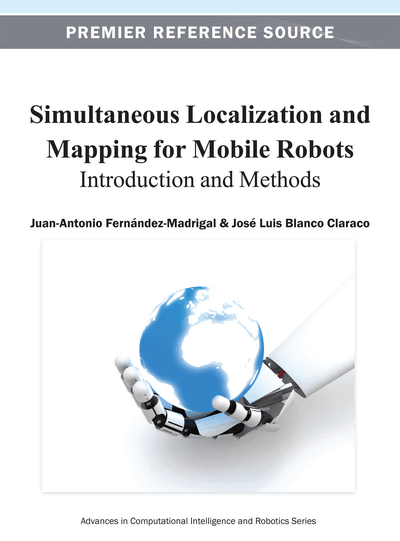# Mobile Robot Localization with Recursive Bayesian Filters

Copyright: © 2013 |Pages: 50
DOI: 10.4018/978-1-4666-2104-6.ch007
OnDemand PDF Download:
Available
\$37.50
No Current Special Offers

## Abstract

In this last chapter of the second section, the authors present probabilistic solutions to mobile robot localization that bring together the recursive filters introduced in chapter 4 and all the components and models already discussed in the preceding chapters. It presents the general, Bayesian framework for a probabilistic solution to localization and mapping. The problem is formally described as a graphical model (in particular a dynamic Bayesian network), and the characteristics that can be exploited to approach it efficiently are elaborated. Among parametric Bayesian estimators, the family of the Kalman filters is introduced with examples and practical applications. Then, the more modern non-parametric filters, mainly particle filters, are explained. Due to the diversity of filters available for localization, comparative tables are included.
Chapter Preview
Top

## Chapter Guideline

• You will learn:

• o

The general structure of the recursive estimation problem underlying mobile robot localization.

• o

The different approaches to instantiate that structure: parametric and non-parametric filters.

• o

The rationale behind the different factors and parameters of a filter for localization.

• o

How to cope with non-linear systems and non-Gaussian random variables.

• o

The pros and cons of each localization filter: how to choose the fittest for your application.

• Provided tools:

• o

Complete formulation and justification of each localization filter.

• o

Tables of computational costs for the presented filtering algorithms.

• Relation to other chapters:

• o

This chapter closes the first part of the book (localization) and sets in motion the basic tools needed for solving the mapping problem.

• o

Modified versions of the filters introduced in this chapter are used for constructing maps in chapter 8 and for SLAM in chapter 9.

Top

## 1. Introduction

Previous chapters have already introduced all the elements required to build a general probabilistic framework for mobile robot localization. Now it is time to glue all the components together to arrive at complete and practical solutions.

Firstly, a word is in order about notation. Up to this point, we have used thenotation in order to highlight the similarities between discrete and continuous distributions (pmfs and pdfs). In contrast, from this chapter on we will switch to the simpler notation(a continuous) and(a discrete), since otherwise the most interesting expressions would always appear as subindices.

The starting point for deriving solutions to localization is the Bayesian inference framework we introduced in chapter 4 for estimating the state of a dynamic system. For convenience, we repeat it here using thenotation:

(1)

As demonstrated in chapter 4, when the system is Markovian and we know the transition function for the system state, this expression can be turned into an incremental form, becoming the base of a sequential or recursive Bayesian filter (MMSE, MED, MAP, etc.):

(2)

Here we can see the role played by the motion (transition) and sensor (likelihood) models discussed in chapters 5 and 6, respectively. Equation 2 is general enough to be applicable to any dynamic Markovian estimation problem, and thus it needs to be instantiated for the particular problems we encounter in mobile robotics. This is the goal of this and the following chapters.

## Complete Chapter List

Search this Book:
Reset